# College Chemistry : Molecules and Compounds

## Example Questions

### Example Question #11 : Intermolecular And Intramolecular Forces

The compound hydrazine is given by the molecular formula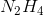. What type of intermolecular forces will govern the behavior of neighboring hydrazine molecules?

Dipole-dipole interaction

Hydrogen bonding

Ion-ion interaction

Dispersion/van der Waals forces

Hydrogen bonding

Explanation:

Hydrazine is a polar compound that possesses the requirements for hydrogen bonding: an "acidic hydrogen" (a hydrogen bonded to a highly electronegative atom such as oxygen, nitrogen or fluorine) and the presence of a lone pair. Both Nitrogen atoms in hydrazine have lone (unshared) electron pairs, and all four hydrogen atoms are "acidic," making hydrazine a candidate for intermolecular hydrogen bonding.

### Example Question #12 : Intermolecular And Intramolecular Forces

List the following three molecules in order from lowest melting point to highest melting point: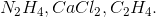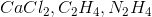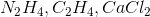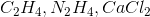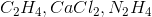Explanation:

Melting point is inversely related to the strength of intermolecular forces between molecules. In other words, the higher the attractive forces between molecules, the harder they will be to pull apart from a solid into a liquid state during melting. Thus, nonpolar compounds experiencing relatively weak intermolecular forces (dispersion/van der Waals forces) tend to have lower melting points than polar compounds experiencing dipole-dipole or hydrogen bonding interactions, and lower still than ionic compounds with strong attractions between positive and negative ionic species. Thus, in this list, the nonpolar compound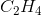has the lowest melting point, followed by the polar compound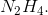The ionic compound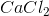has the highest melting point.

### Example Question #1 : Molecular Geometries And Vsepr Theory

What is the molecular geometry of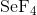?

Square planar

T-shaped

Seesaw

Trigonal pyramidal

Seesaw

Explanation:

Start by drawing the Lewis structure of.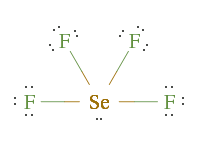Selenium hasfluoride molecules bonded to it, and it has a lone pair. This means its steric number is. A molecule with a steric number ofandlone pair has a seesaw molecular geometry. Note that this is an expanded octet.

### Example Question #2 : Molecular Geometries And Vsepr Theory

What is the molecular geometry of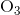?

Linear

Bent

T-shaped

Trigonal planar

Bent

Explanation:

Start by drawing the Lewis structure of.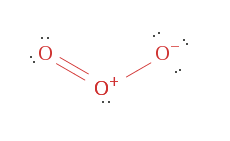Notice that the central oxygen atom hasother oxygen atoms bonded to it, as well as a lone pair. This means it has a steric number of. A molecule with a steric number ofandlone pair has a bent molecular geometry.

### Example Question #3 : Molecular Geometries And Vsepr Theory

What is the molecular geometry of the bonds surrounding the circled atom?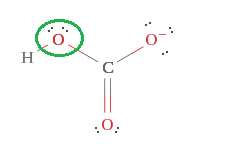T-shaped

Tetrahedral

Bent

Linear

Bent

Explanation:Start by determining the steric number of the circled oxygen atom. Since it is bonded to a hydrogen and a carbon and haslone pairs, its steric number must be. A molecule with a steric number ofandlone pairs has a bent molecular geometry.

### Example Question #4 : Molecular Geometries And Vsepr Theory

What is the molecular geometry of the circled element?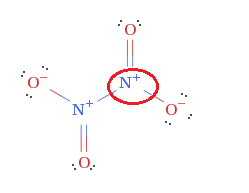Trigonal planar

Trigonal pyramidal

T-shaped

Tetrahedral

Trigonal planar

Explanation:

In order to figure out the molecular geometry of the circled element, first determine the steric number.

The circled nitrogen has three electron groups attached to it, so its steric number is. Since there are no lone pairs on the nitrogen, its electron geometry and its molecular geometry are both trigonal planar.

### Example Question #5 : Molecular Geometries And Vsepr Theory

What is the molecular geometry of the circled element?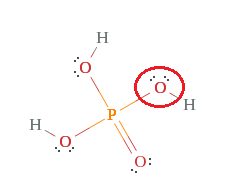T-shaped

Tetrahedral

Bent

Linear

Bent

Explanation:

In order to figure out the molecular geometry of the circled element, first determine the steric number.

Since the circled oxygen has four electron groups attached to it, its steric number is. The circled oxygen also haslone pairs of electrons attached to it; therefore, it has a bent molecular geometry.

### Example Question #6 : Molecular Geometries And Vsepr Theory

Which of the following correctly describes the VSEPR shape of the water molecule,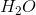?

Trigonal planar

Linear

Trigonal bipyramidal

None of these

Bent

Bent

Explanation:

In the water molecule, there are four electron groups (steric number is four). Two of them (two pairs) are bonded (the bonds between both hydrogen atoms and the oxygen atom), while the other two are lone pairs on oxygen. Thus, the water molecule has a bent shape, with two bonded electron groups and two lone pair electron groups. A linear VSEPR shape has two electron groups, a trigonal planar has three electron groups, and a trigonal bipyramidal shape has five electron groups.

### Example Question #7 : Molecular Geometries And Vsepr Theory

Which of the following is true of Valence Bond theory and Molecular Orbital theory?

The Valence Bond theory states that covalent bonds are formed from atomic orbital overlap while the Molecular Orbital theory is the mathematical combination of atomic orbitals to produce anti bonding and bonding orbitals.

Both Valence Bond theory and Molecular Orbital theory produce hybridized geometries through anti bonding and bonding orbitals.

Neither Valence Bond theory nor Molecular Orbital theory produces hybridized geometries.

The Valence Bond theory is the mathematical combination of atomic orbitals to produce anti bonding and bonding orbitals while the Molecular Orbital theory states that covalent bonds are formed from atomic orbital overlap.

The Valence Bond Theory states that covalent bonds are the result of electron repulsion while the Molecular Orbital theory states that covalent bonds form as a result of atomic overlap.

The Valence Bond theory states that covalent bonds are formed from atomic orbital overlap while the Molecular Orbital theory is the mathematical combination of atomic orbitals to produce anti bonding and bonding orbitals.

Explanation:

The Valence Bond theory states that covalent bonds are formed from atomic orbital overlap while the Molecular Orbital theory is the mathematical combination of atomic orbitals to produce anti bonding and bonding orbitals.

Hybridization occurs through combining atomic orbitals, a concept consistent with Valence Bond theory. Common bonds found in Valence Bond hybridization are sigma and pi bonds which overlap end-to-end or side-to-side respectively. Orbitals are combined in Molecular Orbital theory, producing either bonding or anti bonding orbitals. Molecular Orbital theory shows the destructive or constructive interference of sigma and pi bonds (displayed through bonding and anti bonding orbitals).

### Example Question #8 : Molecular Geometries And Vsepr Theory

A molecule has 4 electron groups and 2 lone pairs. What is the VSEPR notation, electron group geometry, and molecular geometry?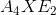, octahedral, square pyramidal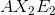, tetrahedral, bent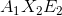, trigonal bipyramidal, t-shaped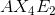, octahedral, square planar, tetrahedral, tetrahedral, tetrahedral, bent
A molecule that has 4 electron groups and 2 lone pairs has an VSEPR notation of:.  Theindicates the central atom, thethe number of bonding atoms, and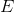the number of lone pairs.  There are 2 bonding atoms and 2 lone pairs, totaling a total of 4 electron groups.  All tetrahedral geometries consist of 4 electron groups. Molecular geometries of electron groups with the tetrahedral are tetrahedral (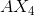), trigonal pyramidal (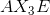), and bent ().
Therefore the correct answer is:, tetrahedral, bent.